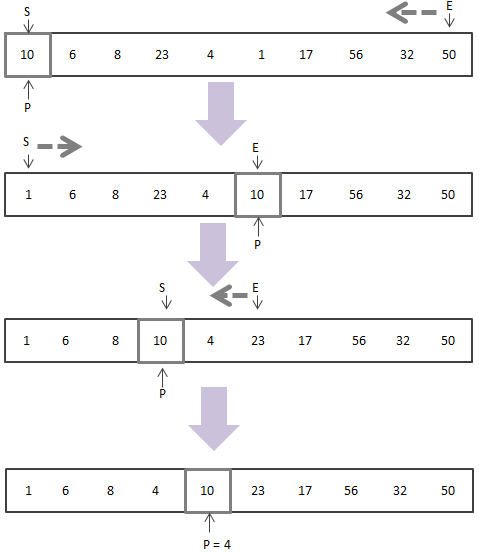# 迹忆客专注技术分享

## 排序算法学习之路——快速排序

1 、从数列中挑出一个元素，称为 “基准”（这个基准的找出方式有很多，在这里我们就默认使用第一个元素作为基准）

2、 重新排序数列，所有元素比基准值小的摆放在基准前面，所有元素比基准值大的摆在基准的后面（相同的数可以到任一边）。在这个分区退出之后，该基准就处于数列的中间位置。这个称为分区（partition）操作。

3、从第二步中得到的基准的中间位置将数组分成两部分，递归地（recursive）把小于基准值元素的子序列和大于基准值元素的子序列排序。

4、重复第二步，直到子序列的数值个数为1``````function FindPv(&\$arr,\$s,\$e){
\$p = \$s; //基准起始位置
\$v = \$arr[\$p];  //将数组的第一个值作为基准值
while(\$s<\$e){
while(\$arr[\$e]>\$v&&\$e>\$p){
\$e--;
}
\$arr[\$p] = \$arr[\$e];
\$p = \$e;
while(\$arr[\$s]<\$v&&\$s<\$p){
\$s++;
}
\$arr[\$p] = \$arr[\$s];
\$p = \$s;
}
\$arr[\$p] = \$v;
return \$p;
}
function PvSort(&\$arr,\$s,\$e){
if(\$s>=\$e) return ;
\$nextP = FindPv(\$arr,\$s,\$e);  //找到下一个基准所在位置
PvSort(\$arr,\$s,\$nextP-1);
PvSort(\$arr,\$nextP+1,\$e);
}
\$arr = array(10,6,8,23,4,1,17,56,32,50,11,9);
PvSort(\$arr);
print_r(\$arr);
``````

## 相关文章

### 在 Scala 中对数组进行排序## 热门标签